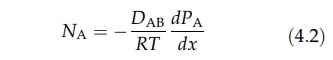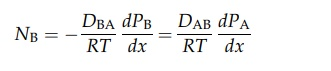# Equimolecular Counter Diffusion

| Home | | Pharmaceutical Technology |

## Chapter: Pharmaceutical Engineering: Mass Transfer

Pharmaceutical Engineering : Mass Transfer - Equimolecular Counter Diffusion

EQUIMOLECULAR COUNTERDIFFUSION

If no bulk flow occurs in the element of length dx, shown in Figure 4.1, the rates of diffusion of the two gases, A and B, must be equal and opposite, that is,

NA= - NB

The partial pressure of A changes by dPA over the distance dx. Similarly, the partial pressure of B changes by dPB. Since there is no difference in total pressure across the element (no bulk flow), dPA/dx must equal -dPB/dx. For an ideal gas, the partial pressure is related to the molar concentration by the relation

PAV = nART

where nA is the number of moles of gas A in a volume V. Since the molar concentration, CA, is equal to nA/V,

PA = CART

Therefore, for gas A, equation (4.1) can be written aswhere DAB is the diffusivity of A in B. Similarly,It therefore follows that DAB = DBA = D. If the partial pressure of A at x1 is PA1 and that at x2 is PA2, integration of equation (4.2) givesA similar equation may be derived for the counterdiffusion of gas B.